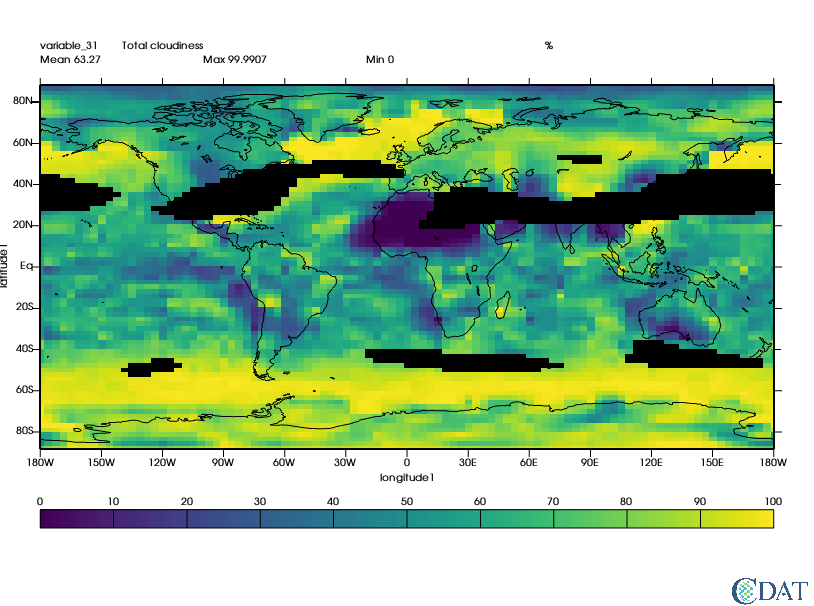The CDAT software was developed by LLNL. This tutorial was written by Charles Doutriaux. This work was performed under the auspices of the U.S. Department of Energy by Lawrence Livermore National Laboratory under Contract DE-AC52-07NA27344.

## Preparing the Notebook¶

In :
import requests
r = requests.get("https://cdat.llnl.gov/cdat/sample_data/clt.nc",stream=True)
with open("clt.nc","wb") as f:
for chunk in r.iter_content(chunk_size=1024):
if chunk:  # filter local_filename keep-alive new chunks
f.write(chunk)

import cdms2
f = cdms2.open("clt.nc")
clt = f("clt", time=slice(0,1), squeeze=1) # Get first month
u = f("u", level=slice(0,1), squeeze=1)
v = f("v", level=slice(0,1), squeeze=1)
clt = clt.regrid(u.getGrid(), regridTool="regrid2") # Put data on same grid

# computes wind speed
import MV2
speed = MV2.sqrt(u**2+v**2)
print("Max speed:", speed.max())
print("Mean speed:",speed.mean())
print("Min speed:",speed.min())

# Prepare graphics
import vcs
x=vcs.init()

Max speed: 68.91321
Mean speed: 16.25912330863402
Min speed: 0.061108682


In :
# Let's mask out area where wind speed is greater than twice meanOut:
<vcs.displayplot.Dp at 0x7fbcc96a2380>

### Generating a Land-sea Mask on any grid¶

Conveniently CDAT can generate masks for you (for regular grids only).

The observed data set used here as the basis for creating realistic model land/sea masks was obtained from the U.S. Navy on a 1/6 degree longitude-latitude grid.

More on the technique used can be read here

In [ ]:
import cdutil
x.clear()


### Surface type by region masks¶

CDAT also provide capabilities to mask regions. Original regions and their numbers come from EzGet

The function requires both a land/sea mask and a file reporting "regions", default "region" mask is as follow:Regions tables is:In [ ]:
regions, guess = cdutil.generateSurfaceTypeByRegionMask(mask2*100., verbose=False)

In [ ]:
# let's take a look
x.clear()
x.plot(regions)

In [ ]:
# Now let's extract the indian ocean which according to table are area 205 and 206
ind1 = MV2.equal(regions,205)
ind2 = MV2.equal(regions,206)
indian_ocean = MV2.logical_or(ind1,ind2)